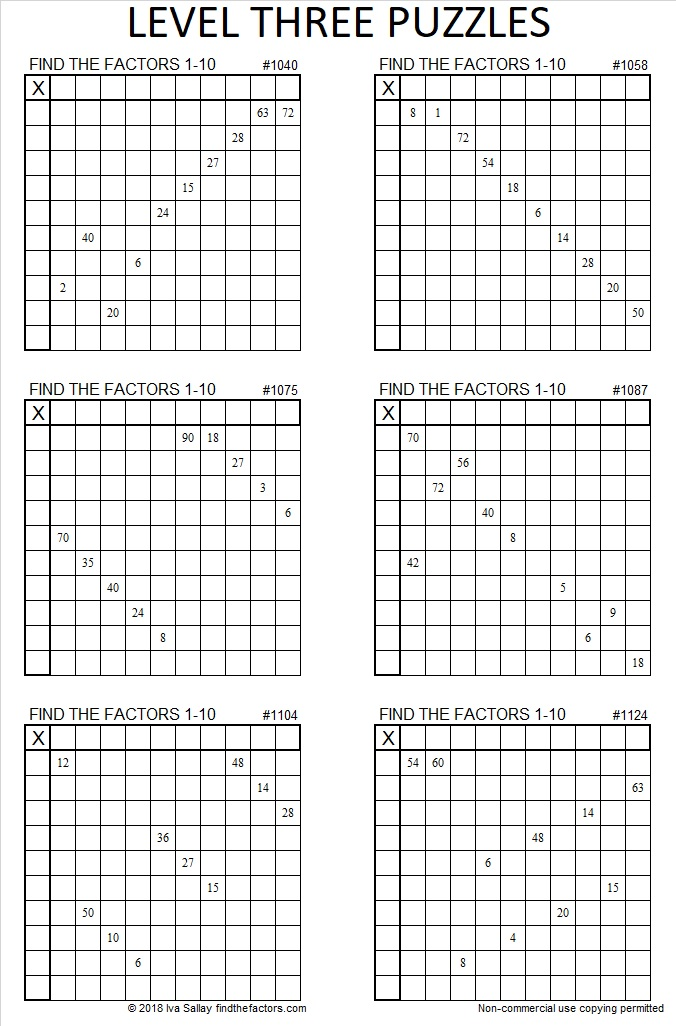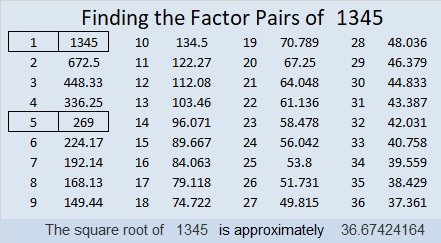# 1345 Level Three Puzzles Only

To solve a level 3 puzzle, start with a clue in the top row of the puzzle and find one other clue in the same row or column. Write the factors for those two clues in the appropriate cells and then work your way down the puzzle cell by cell until you have written the numbers from 1 to 10 in both the first column and the top row.Level 3 puzzles are a jump from level 2, but still significantly easier than any of the higher level puzzles, because the needed clues are kept in a logical order from the top of the puzzle to the bottom. You can print the puzzles above and work on them or you can access the whole collection in an excel sheet: Level 3’s from 2018. Print them or type the answers directly in excel.

Now I’ll share some information about the number 1345:

• 1345 is a composite number.
• Prime factorization: 1345 = 5 × 269
• 1345 has no exponents greater than 1 in its prime factorization, so √1345 cannot be simplified.
• The exponents in the prime factorization are 1, and 1. Adding one to each exponent and multiplying we get (1 + 1)(1 + 1) = 2 × 2 = 4. Therefore 1345 has exactly 4 factors.
• The factors of 1345 are outlined with their factor pair partners in the graphic below.1345 is the sum of two squares in two different ways:
36² + 7² = 1345
33² + 16² = 1345

1345 is also the hypotenuse of FOUR Pythagorean triples:
345-1300-1345 which is 5 times (69-260-269)
504-1247-1345 calculated from 2(36)(7), 36² – 7², 36² + 7²
807-1076-1345 which is (3-4-5) times 269
833-1056-1345 calculated from 33² – 16², 2(33)(16), 33² + 16²

This site uses Akismet to reduce spam. Learn how your comment data is processed.